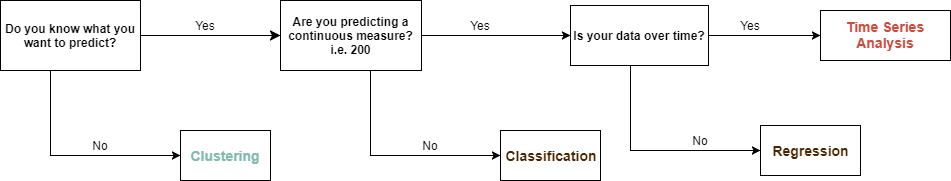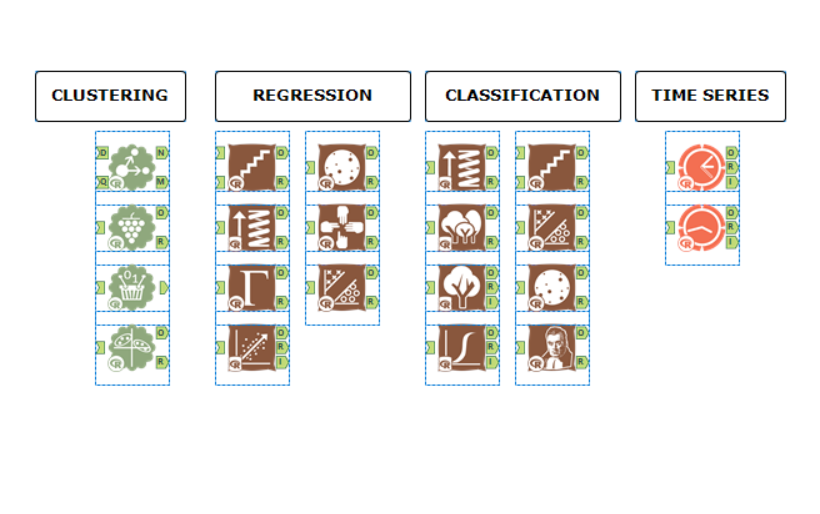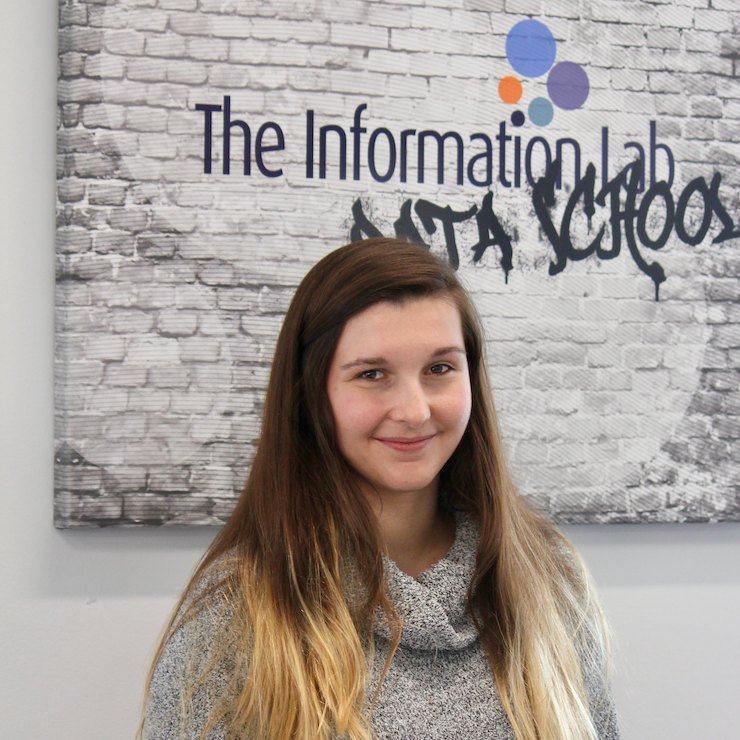## Alteryx Analytics: Intro to Predictive Model Selection

by Mina Ozgen

### Regression, Classification, Clustering or Time Series?

Want to create a predictive model in Alteryx but not sure what type of model you are supposed to use? Models are dependant on their assumptions and are limited by their process and output. When first deciding the model type you have to make a few assessments.

The following flow chart determines what kind of modelling you should perform based on your data and the analysis you are performing.If you do decide to deviate from the above diagram the model will do one or more of the following:

1. Fail
2. Produce a terrible prediction
3. Be statistically invalid

### Methods

Within each of these “kinds” of modelling, there are numerous individual methods.

Model selection will be down to the nature of the data and nature of the analysis. Factors such as the distribution of data will decide if for example a K-Medians should be used over a K-Means (if the distribution is skewed then K-Medians is a better methodology); or if Gamma Regression or Count Regression should be used over Linear Regression. Another example would be if you know that your time series data also contains important explanatory variables you would prefer ARIMA with covariates over ETS. My final example would be if you are trying to solve a classification problem with 3 classes: high, medium, low; this would be unsuitable for logistic regression who’s purpose is to solve binary (two possibilities) classification.

There are numerous nuances to the methodologies and Alteryx’s documentation attempts to add a bit of context for each. However, if you really want to invest into a model for the use I would highly recommend reading more in-depth descriptions of the models and their assumptions to verify if it is the right fit with your data. There is often the temptation in predictive analysis with Alteryx to simply accept the most accurate model after one test using a comparison tool. However, this may end up not being robust. When the behaviour of the model and the structure of the data are fundamentally opposing, the correlation the predictive values have with a forecast (part of how the selection is made) cannot be attributed to true explanation; these could deviate at any point “without reason”. In simpler terms, what the model is able to predict with the current data set may never have near the same accuracy in subsequent uses of the model.

Currently, in Alteryx, there are tools for the following methods:

#### Clustering

K-Centroid Analysis (K-Mean, K-Median)
Nearest Neighbors
Principal Components
MB Affinity

#### Classification

Logistic Regression
Decision Tree
Forest Model
Boosted Model
Spline Model
Stepwise
Support Vector Machine
Naive Bayes Classifier

#### Regression

Linear Regression
Boosted Model
Spline Mode
Stepwise
Gamma Regression
Count Regression
Support Vector Machine

#### Time Series

ARIMA
ETSIf you have any questions on model selection don’t be afraid to ask, I will endeavour to either answer your question or direct you to good material to assist you.Mina Ozgen

Mon 02 Oct 2017

Thu 24 Aug 2017

Wed 16 Aug 2017

Tue 15 Aug 2017